# TSA Skewness and Kurtosis VI

LabVIEW 2014 Advanced Signal Processing Toolkit Help

Edition Date: June 2014

Part Number: 372656C-01

»View Product InfoDownload Help (Windows Only)

Owning Palette: Statistical Analysis VIs

Computes the skewness and kurtosis values of a univariate or multivariate (vector) time series. Wire data to the Xt input to determine the polymorphic instance to use or manually select the instance.

Use the pull-down menu to select an instance of this VI.

 Select an instance TSA Skewness and Kurtosis (Waveform)TSA Skewness and Kurtosis (Array)TSA Vector Skewness and Kurtosis (Waveform)TSA Vector Skewness and Kurtosis (Array)

## TSA Skewness and Kurtosis (Waveform)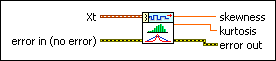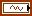Xt specifies the univariate time series.error in describes error conditions that occur before this node runs. This input provides standard error in functionality.skewness returns the skewness value of the input time series.kurtosis returns the kurtosis value of the input time series.error out contains error information. This output provides standard error out functionality.

## TSA Skewness and Kurtosis (Array)Xt specifies the univariate time series.error in describes error conditions that occur before this node runs. This input provides standard error in functionality.skewness returns the skewness value of the input time series.kurtosis returns the kurtosis value of the input time series.error out contains error information. This output provides standard error out functionality.

## TSA Vector Skewness and Kurtosis (Waveform)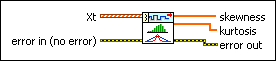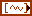Xt specifies the multivariate (vector) time series.error in describes error conditions that occur before this node runs. This input provides standard error in functionality.skewness returns the vector skewness values of the multivariate (vector) time series.kurtosis returns the vector kurtosis values of the multivariate (vector) time series.error out contains error information. This output provides standard error out functionality.

## TSA Vector Skewness and Kurtosis (Array)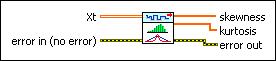Xt specifies the multivariate (vector) time series. Each column of the 2D array represents a vector at certain time.error in describes error conditions that occur before this node runs. This input provides standard error in functionality.skewness returns the vector skewness values of the multivariate (vector) time series.kurtosis returns the vector kurtosis values of the multivariate (vector) time series.error out contains error information. This output provides standard error out functionality.

## TSA Skewness and Kurtosis Details

This VI calculates the skewness value according to the following equation: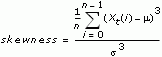where n is the number of samples of the input time series Xt, m is the arithmetic mean of Xt, and s is the standard deviation of Xt. Skewness is a symmetry measurement of the time series distribution. Negative values indicate left skewness. Positive values indicate right skewness.

This VI calculates the kurtosis value according to the following equation:where n is the number of samples of the input time series Xt, m is the arithmetic mean value of Xt, and s is the standard deviation of Xt. Kurtosis is a peakedness measurement of the time series distribution. Kurtosis values close to 3 indicate normal-peak distribution. Kurtosis values less than 3 indicate a flatter distribution than normal distribution. Kurtosis values greater than 3 indicate a sharper distribution than normal distribution.

## Example

Refer to the Series Statistical Analysis VI in the labview\examples\Time Series Analysis\TSAGettingStarted directory for an example of using the TSA Skewness and Kurtosis VI.